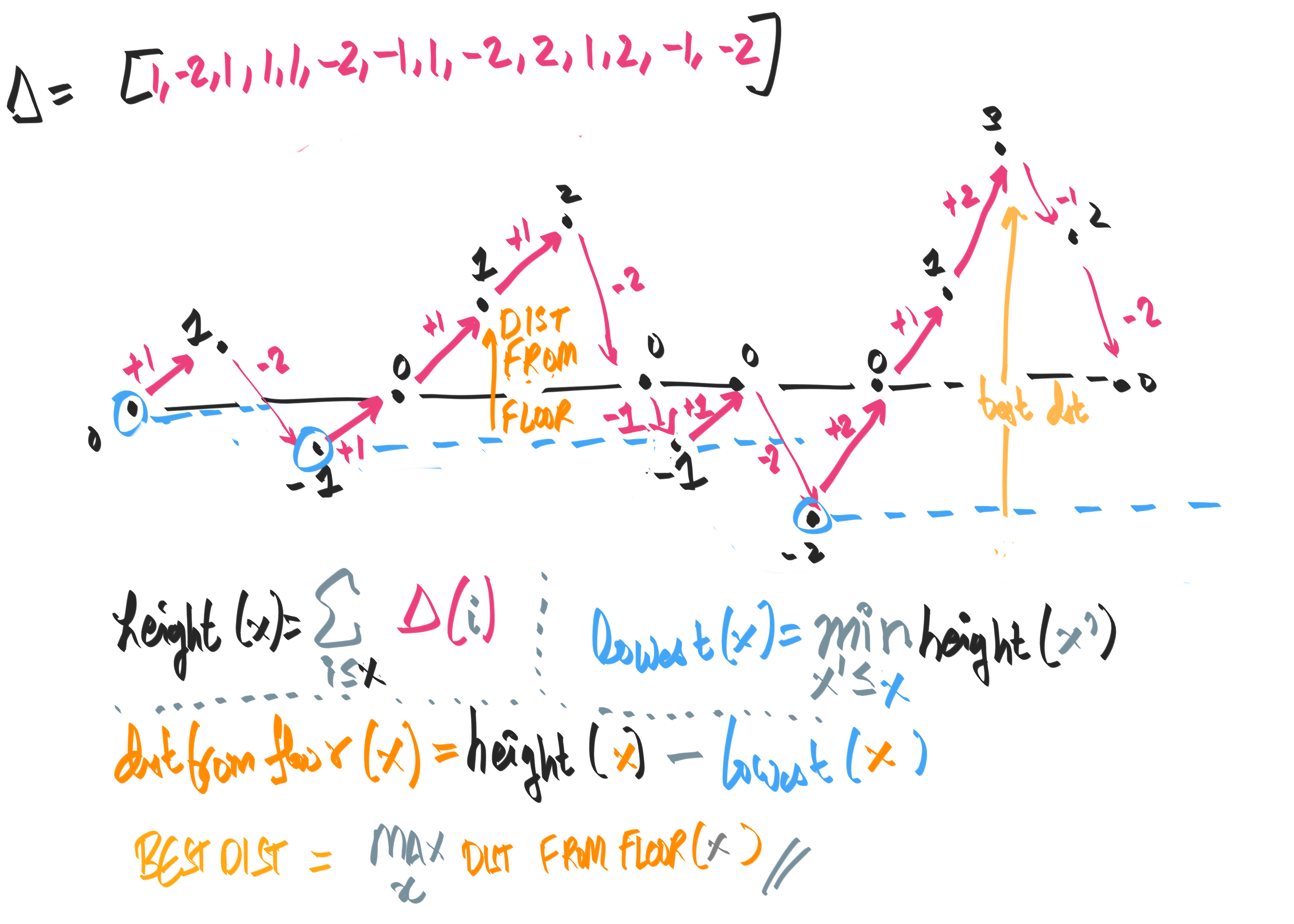## § Parallelisable version of maximum sum subarray

I learnt of a "prefix sum/min" based formulation from the solution to question D, codeforces educational round 88 . The idea is to start with the max prefix sum $opt$ (for optima) as the difference of right minus left:
\begin{aligned} &opt \equiv \max_{(L, R)}: \sum_{L \leq i \leq R} a[i] \\ &= \max_R: \left(\sum_{0 \leq i \leq R} a[i] - \min_{L \leq R}: \sum_{0 \leq i \leq L \leq R} a[i] \right) \\ \end{aligned}
Which is then expressed as:
\begin{aligned} &asum[n] \equiv \sum_{0 \leq i \leq n} a[i] \\ &opt = \max_R: (asum[R] - \min_{(L \leq R)}: asum[L]) \end{aligned}
\begin{aligned} &aminsum[n] \equiv \min_{0\leq i \leq n} asum[i] \\ &opt = \max_R: (asum[R] - aminsum[R]) \end{aligned}
Since $asum$ is a prefix-sum of $a$, and $amin$ is a prefix min of $asum$, the whole thing is $O(n)$ serial, $O(\log n)$ parallel. In haskell, this translates to:
let heights deltas = scanl (+) 0 deltas
let lowest_heights = scanl1 min . sums
let elevations xs = zipWith (-) (sums xs) (lowest_heights xs)
-- elevations [1, 2, 3, -2, -1, -4, 4, 6]
-- > [0,1,3,6,4,3,0,4,10]
let max_elevation deltas = max (elevation deltas)
best = max_elevations [1, 2, 3, -2, -1, -4, 4, 6]

lowest_heights keeps track of the sea level, while the elevations computes the elevation from the lowest height. The maximum sum subarray will correspond to treating the elements of the array as deltas, where we are trying to find the highest elevation. since elevation is an integral (sum) of the deltas in height.